# Chemical kinetics   Share

## What is Chemical kinetics

“You can’t cross the sea merely by standing and staring at the water.” – Tagore

Chemical kinetics is the branch of chemistry which deals majorly with the rates of chemical reactions. There are many reactions which occur instantaneously like AgNO3 with HCl to form a salt i.e, AgCl while there are many reactions which occur too slow like the conversion of diamond into graphite. Along with feasibility, there are various other factors like rate and other parameters which control the rate of reactions. Chemical kinetics consider into account of all such types of reactions and tell us about their rate and the order of these reactions. In this chapter, you will learn about the rates and order of the reactions, Arrhenius equation, collision theory of reactions, etc.

In everyday life, there are various important chemical reactions which occur around us as follows:

• Role of chemical kinetics in the "refrigerator": We keep our food items in the refrigerator because it lowers the temperature of these substances. As the temperature is lowered, the reaction rates are low and thus the food items can be used for longer times.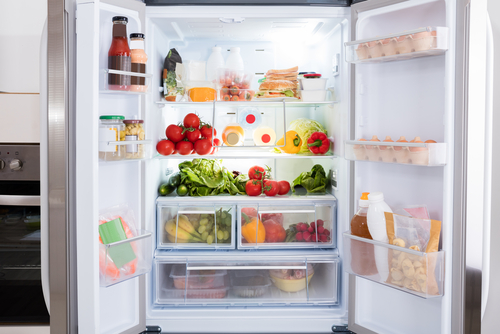• Role of chemical kinetics in the making of "popcorn": Each popcorn kernel contains little amount of water in them. Now when the popcorn kernels are heated, then the water turns into the vapour which tries to come out of the kernel shell. Due to this heating and vapourisation, as the pressure is further is increased, the kernel shells are burst out with a 'pop' sound.

Prepare Online for JEE Main/NEET

Crack JEE 2021 with JEE/NEET Online Preparation Program

Prepare Online for JEE Main/NEET

Crack JEE 2021 with JEE/NEET Online Preparation Program• In chemical kinetics, you also learn about how on changing the reaction conditions like temperature and pressure, the rate of reaction changes. It also gives you information about the reaction mechanisms, activation energy and reaction intermediates.

## Notes for Chemical Kinetics

In this section, you will study about the important topics of the chapter, overview, formulae and some important tips and guidelines for the preparation of the chapter at the best.

## Important Topics of Chemical Kinetics

• Rate of chemical reaction

• Factors influencing the rate of reaction

• Order of a reaction

• Zero-order reactions

• First order reactions

• Second order reactions

• Pseudo first order reactions

• Arrhenius equation, Activation energy and its determination

• Collision theory of reactions

## Overview of the Chemical Kinetics

(i) Rate of reaction: Similar to any object moving with a certain velocity, chemical reactions also occur with certain velocities also known as the rate of reaction. This rate of reaction is defined as how fast the product is forming or how fast the reactants are getting consumed with respect to time. Mathematically, it can be expressed as follows:(ii) Rate expression and rate constant: For the general reaction:

The rate equation is given as follows:

This equation can be written as follows:

where x and y may or may not be stoichiometric coefficients. The addition of these components (x+y) gives the order of the reaction.

(iii) Order of the reaction: The rate equation of a chemical reaction is given as:

where (x+y) gives the order of the reaction and k is the rate constant.

• Units of rate constant: For a general reaction:

The unit of rate constant (k) depends on the order of the reaction i.e. (x+y).

 Reaction type Order of reaction Units of rate constant Zero-order reaction 0 mol L-1 s-1 First order reaction 1 s1 Second order reaction 2 mol-1L s-1
• Zero-order reaction: It is the type of reaction, where the order of reaction is proportional to the zero power of the concentration of reactants. The rate equation is given as follows: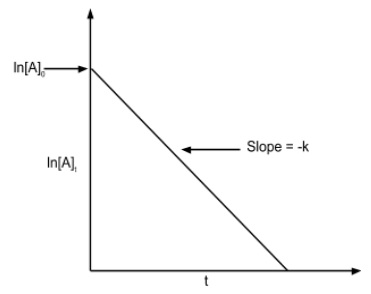• First order reaction: In these type of reactions, the rate of reaction is proportional to the first power of the concentration of reactants. The rate equation is given as follows: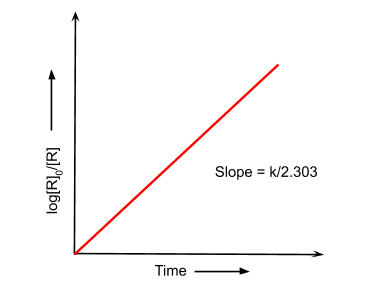• The half-life of reaction: It is the time at which the concentration of reactant is half of its initial value. It is denoted by t1/2. Mathematically, it can be described as follows:

• Arrhenius Equation: The rate of a chemical reaction depends on the temperature. For every 100 rise in temperature, the rate constant gets doubled. This temperature dependency of the rate of chemical reaction is explained by the Arrhenius equation as given below:
k = A e-Ea/RT
where A is the Arrhenius factor, Ea is the activation energy and R is the gas constant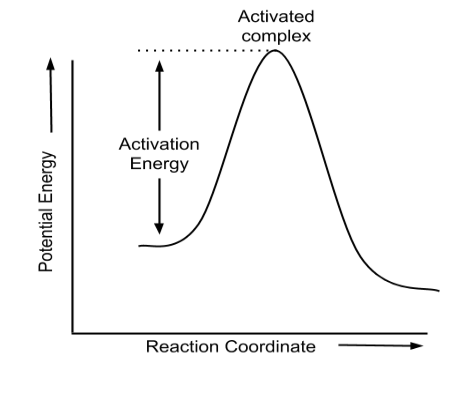• Collision Theory of Chemical Reactions: This theory gives a deeper insight into the mechanism of the chemical reactions. According to this theory, the reactant molecules are considered to be spherical molecules and the reaction occurs when these spherical molecules collide with each other. Mathematically, it can be described as follows:
Rate = ZAB eEa/RT
where ZAB is the collision frequency. The number of collisions per second per unit volume of the reaction mixture is known as "collision frequency(Z)".

## How to prepare for Chemical Kinetics?

• This chapter is a part of physical chemistry. There are some simple formulas and equations like zero order reaction, first-order reaction, etc. which you must understand completely because these concepts are the root of this chapter.

•  Before reading this chapter, first, you must have the basic knowledge of the mole concept.

• You must deeply observe how the graphs show some trends, how to find the rate constants from the graphs, how to find the order of the reactions, etc.

• Rest this chapter is very simple, just be regular and be consistent in your numerical practice.

## Prescribed Books for Chemical Kinetics

First, you must finish the class XII NCERT textbook and solve each and every example and unsolved question given in it. Then for advanced level preparation like JEE and NEET, you must follow R.C. Mukherjee and O.P. Tandon. You must definitely solve the previous year papers. Meanwhile, in the preparation, you must continuously write the mock tests for the depth of knowledge. This article will help you to provide with the variety of questions for deeper knowledge with the help of videos, articles and mock tests.

## Chemistry Chapter- wise Notes for Engineering and Medical Exams

 Chapters No. Chapters Name Chapter 1 Some basic concepts in chemistry Chapter 2 States of matter Chapter 3 Atomic Structure Chapter 4 Solutions Chapter 5 Chemical Thermodynamics Chapter 6 Equilibrium Chapter 7 Redox Reaction and Electrochemistry Chapter 9 Surface Chemistry Chapter 10 General Principle and processes of Isolation of metals Chapter 11 Classification of Elements and Periodic table Chapter 12 Hydrogen Chapter 13 p- block Elements Chapter 14 s-block Elements (Alkali and Alkaline Earth Metals) Chapter 15 Chemical Bonding and Molecular Structure Chapter 16 d- and f- BLOCK ELEMENTS Chapter 17 Coordination Compounds Chapter  18 Environmental Chemistry Chapter 19 Purification and Characterisation of Organic Compounds Chapter 20 Some Basic Principles of Organic Chemistry Chapter 21 Hydrocarbons Chapter 22 Organic Compounds containing Halogens Chapter 23 Organic Compounds containing Oxygen Chapter 24 Organic Compounds Containing Nitrogen Chapter 25 Polymers Chapter 26 Biomolecules Chapter 27 Chemistry in Everyday Life Chapter 28 Principles Related to Practical Chemistry

### Topics from Chemical kinetics

• Rate of a chemical reaction ( JEE Main ) (5 concepts)
• rate law, rate constant and its units ( JEE Main ) (3 concepts)
• factors affecting the rate of reactions: concentration, temperature, pressure and catalyst ( JEE Main ) (6 concepts)
• effect of temperature on rate of reactions -Arrhenius theory, activation energy and its calculation ( JEE Main ) (8 concepts)
• order and molecularity of reactions ( JEE Main ) (6 concepts)
• elementary and complex reactions ( JEE Main ) (1 concepts)
• collision theory of bimolecular gaseous reactions ( JEE Main ) (3 concepts)
• their characteristics and half -lives ( JEE Main ) (5 concepts)
• RATE OF A CHEMICAL REACTION ( JEE Main ) (3 concepts)
• FACTORS INFLUENCING RATE OF A REACTION ( JEE Main ) (4 concepts)
• INTEGRATED RATE EQUATIONS ( JEE Main ) (21 concepts)
• TEMPERATURE DEPENDENCE OF THE RATE OF A REACTION ( JEE Main ) (9 concepts)
• RADIOACTIVITY ( JEE Main ) (6 concepts)

### Important Books for Chemical kinetics

•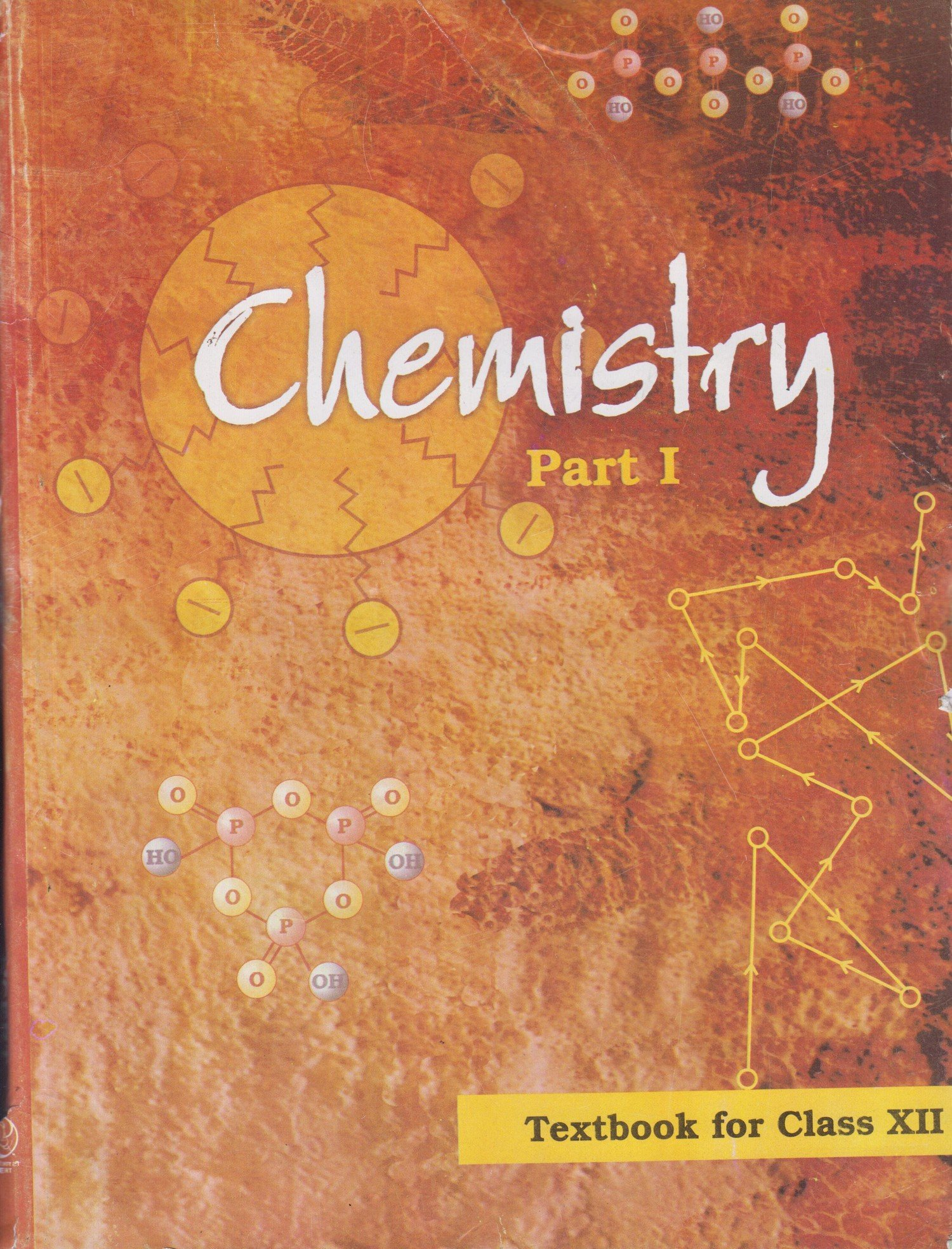•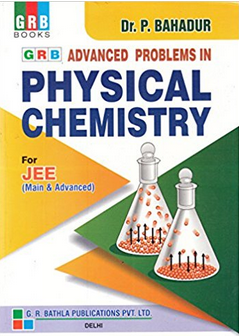Exams
Articles
Questions